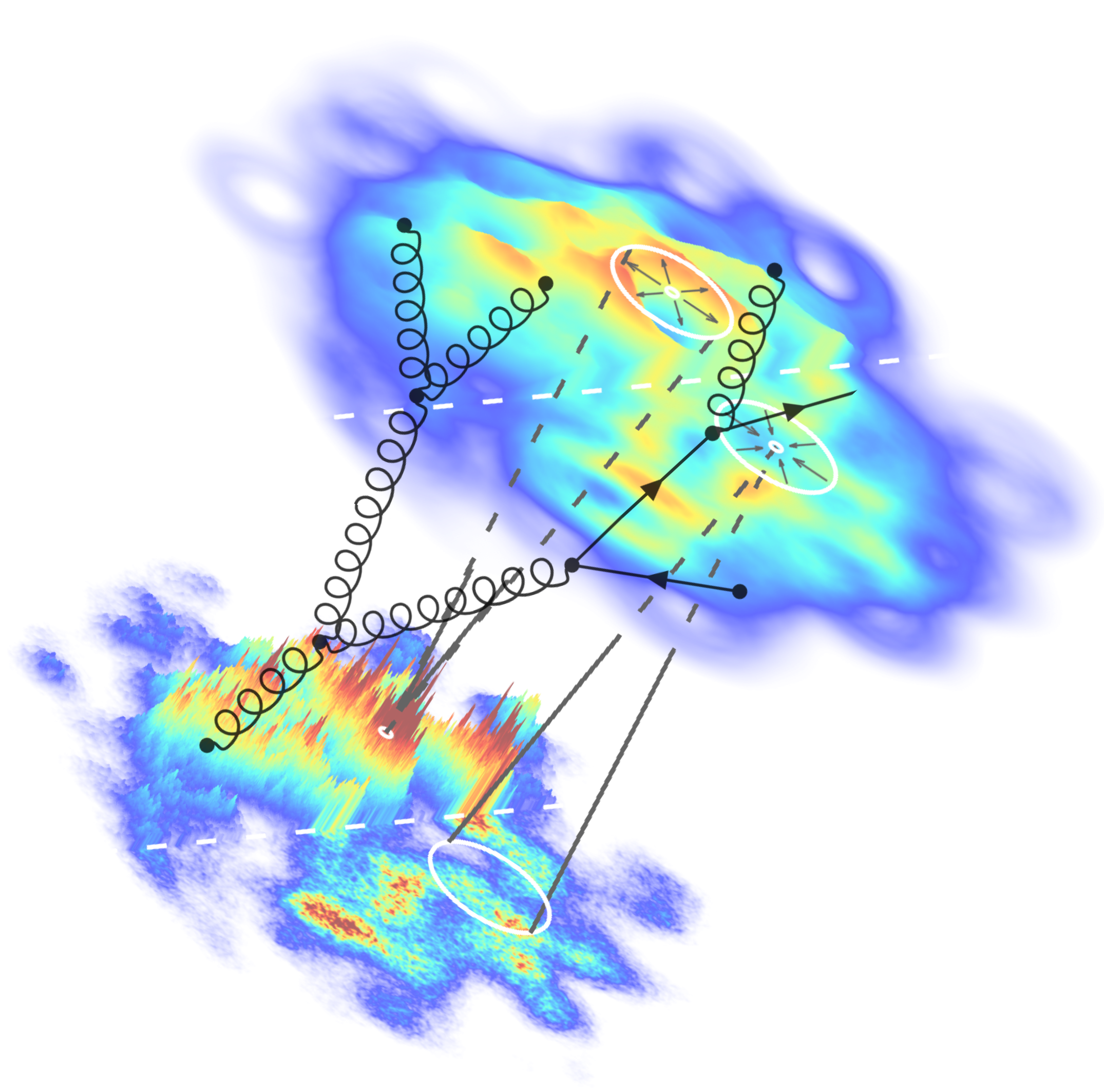# NON-EQUILIBRIUM PHYSICS WS 20/21

Lectures: Mon 10:00-12:00; Wed 12:00-14:00 (ONLINE)
Tutorials: Mon 12:00-14:00 (ONLINE)

Instructor: Jun.-Prof. Dr. Soeren Schlichting
(sschlichting AT physik.uni-bielefeld.de)

Tutor: Ismail Soudi
(isma AT physik.uni-bielefeld.de)

This graduate (M.Sc.) level course provides an introduction to non-equilibrium statistical physics, based on the book of N. Pottier on ``Nonequilibrium Statistical Physics`` and the lecture notes of Prof. Dr. Nicolas Borghini available on his webpage .

.

Due to the COVID-19 situation, lectures will be recorded and uploaded on a weekly basis. Notes from the lectures will be posted on this website. Weekly discussion sessions and tutorials will be held via ZOOM; the respective link will we provided to registered participants via E-Mail. Questions regarding the lectures and tutorials can also be posted in the EKVV Lernraum Forum and shall be addressed during the weekly discussions (lectures) or answered directly in the Forum (tutorials).

Topics include:

- Thermodynamics of irreversible processes
- Statistical description of non-eq. systems
- Kinetic theory
- Weak wave turbulence
- Stochastic processes
- Linear repsonse theory

Evaluation of > Lectures and Tutorials

Exercise sheets:

Course materials:

Introductory remarks
Notes from Introduction

Week 1 -- Thermodynamics of irreversible processes: Equilibrium thermodynamics (reminder), Description of irreversible processes, Local equilibrium, Entropy production in discrete & continuous systems
Notes from Lecture 1 and Notes from Lecture 2

Week 2 -- Thermodynamics of irreversible processes: Linear irreversible proccesses, Simple transport phenomena, Curie and Onsager relations
Notes from Lecture 3 and Notes from Lecture 4

Week 3 -- Thermodynamics of irreversible processes: Hydrodynamics, Navier-Stokes equation
Notes from Lecture 5 and Notes from Lecture 6

Week 4 -- Thermodynamics of irreversible processes: Simple problems in Hydrodynamics
Notes from Lecture 7
-- Statistical description of non-equilibrium systems: Classical systems
Notes from Lecture 8

Week 5 -- Statistical description of non-equilibrium systems: Quantum systems, Wigner-Weyl formalism, Reduced distributions, BBGKY Hierarchy
Notes from Lecture 9 and Notes from Lecture 10
Some additional LaTeX Notes on Wigner-Weyl formalism

Week 6 -- Statistical description of non-equilibrium systems: Vlasov equatiom, Scales in BBGKY Hierarchy
Notes from Lecture 11
-- Kinetic theory: Boltzmann equation, Heuristic derivation Notes from Lecture 12

Week 7 -- Kinetic therory: Boltzmann equation from BBGYKY hierarchy, Balance equations
Notes from Lecture 13 and Notes from Lecture 14

Week 8 -- Kinetic therory: Boltzmann entropy, H-Theorem, Equilibrium solutions, Hilbert expansion
Notes from Lecture 15 and Notes from Lecture 16

Week 9 -- Kinetic therory: Calculation of transport coefficients
Notes from Lecture 17 and Notes from Lecture 18

Week 10 -- Kinetic therory: Hydrodynamics from kinetic theory
Notes from Lecture 19
-- Wave turbulence: Stationary turbulence, Richardson cascade, Kolmogorov-Zhakarov spectra
Notes from Lecture 20
See also textbook V.E. Zakharov, V.S. L'vov and G. Falkovich; Kolmogorov Spectra of Turbulence I: Wave turbulence (Chapters 1,2,3)

Week 11 -- Wave turbulence: Energy flux along the cascade, Decaying turbulence
Notes from Lecture 21 and Notes from Lecture 22

Week 12 -- Stochastic processes: Stochastic Differential Equations, Brownian Motion
Notes from Lecture 23 and Notes from Lecture 24

Week 13 -- Stochastic processes: Markov Processes, Fokker-Planck Equation
Notes from Lecture 25 and Notes from Lecture 26

Week 14 -- Linear response theory: Kubo relation, Equilibrium correlation functions
Notes from Lecture 27 and Notes from Lecture 28